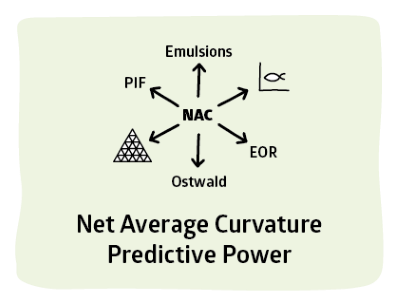## Net Average Curvature (NAC)

### Quick Start

HLD is powerful, but limited. When coupled with NAC then most of the interesting things seen in other apps (such as IFT and Solubility calculations) become possible.

People are rather nervous about NAC. But as you will see, the idea is very simple. The exciting thing is that so much good stuff follows from such elegant simplicity.The Basics section quickly skipped over the theory of NAC, Net Average Curvature. When there are pure oil drops in water the curvature (1/radius) is easy to calculate because the radius of the drop can be calculated using some basic facts about the size and amount of the surfactant. Similarly pure water drops in oil can be calculated. And the continuous phase can also produce a pseudo-radius which is 3*Vol/Head_Area. The difference between the two curvatures is the Net Curvature

Net Curvature= 1/Roil-1/Rwater

This Net Curvature happens to equal HLD/Tail_Length. So we are close to being able to calculate the volumes of both phases from the Net Curvature via the HLD and the known Tail_Length. Unfortunately when HLD=0 the formula tells us that the mutual solubility (proportional to Tail_Length/HLD) is infinite, which is clearly impossible. That's why we need the Average Curvature:

Average Curvature = 0.5*(1/Roil+1/Rwater).

The Average Curvature turns out to be equal to 1/ξ, the de Gennes Coherence Length which is a parameter than can be measured for a surfactant/oil mix via neutron scattering or by measuring phase volumes. The fact that the AC is finite (limited to 1/ξ) limits the radii so the calculated solubilities are always finite.

Calculations then take into account both the Net Curvature and the Average Curvature so the theory is called Net Average Curvature, NAC.

The Tail Length, L is in turn estimated from the extended tail length, Lc via

L~1.3Lc

(actually 1.2 for ionics and 1.4 for ethoxylates and, for simplicity, 1.3 for "others")

and Lc is calculated from the number of carbons in the chain via the Tanford equation:

Lc = 1.5 + 1.265nc

Finally, nc is simply AC, the number of alkane carbons for a pure linear alkane or, it depends on the number of Branched Carbons, BC, the number of EO or PO within the tail, NO, the number of benzenes, NB, and the number of o-Xylenes, NX. The formulae are a little vague, but the following is one version:

nc = AC + 0.5BC + 2NO + 3.5NB + 2.5NX

A later email from an expert, Sanja Natali, mentioned that carbons on C2 act surprisingly strongly, giving the more sophisticated equation used in the more recent version of the app, using BC2 for the branches at C2 and BMC for mid chain branches:

nc = AC + 6.9BC2 + 0.71BMC + 2NO + 3.5NB + 2.5NX

The calculations are done for you:

### Parameter Calculations

AC
BC2
BMC
NO
NB
NX
nc
Lc Å
L Å

NAC is used in many sections (such as the Fish diagrams) but in this section, the power of NAC is explicitly revealed, first via its ability to calculated drop sizes and, therefore, viscosities, second via a more detailed look at ξ and thirdly via a predictive model of emulsion stability.

Although Practical Surfactants is a joint effort, this NAC section represents the state-of-the-art from the Acosta team. Some of the work shown here has not yet been published and can therefore be considered as being open to refinement. One reason for showing the ideas within Practical Surfactants is to elicit comment, positive or negative (both are welcome!) from the expert surfactant community. If you would like to interact on these NAC issues, please contact Prof Acosta directly at edgar.acosta@utoronto.ca